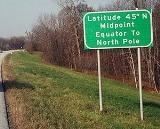xLatitudeOverview

In geography
Geography
Geography is the science that studies the lands, features, inhabitants, and phenomena of Earth. A literal translation would be "to describe or write about the Earth". The first person to use the word "geography" was Eratosthenes...

, the latitude of a location on the Earth
Earth
Earth is the third planet from the Sun, and the densest and fifth-largest of the eight planets in the Solar System. It is also the largest of the Solar System's four terrestrial planets...

is the angular distance
Angular distance
In mathematics and all natural sciences , the angular distance between two point objects, as observed from a location different from either of these objects, is the size of the angle between the two directions originating from...

of that location south or north of the Equator
Equator
An equator is the intersection of a sphere's surface with the plane perpendicular to the sphere's axis of rotation and containing the sphere's center of mass....

. The latitude is an angle
Angle
In geometry, an angle is the figure formed by two rays sharing a common endpoint, called the vertex of the angle.Angles are usually presumed to be in a Euclidean plane with the circle taken for standard with regard to direction. In fact, an angle is frequently viewed as a measure of an circular arc...

, and is usually measured in degree
Degree (angle)
A degree , usually denoted by ° , is a measurement of plane angle, representing 1⁄360 of a full rotation; one degree is equivalent to π/180 radians...

s (marked with °). The equator has a latitude of 0°, the North pole
North Pole
The North Pole, also known as the Geographic North Pole or Terrestrial North Pole, is, subject to the caveats explained below, defined as the point in the northern hemisphere where the Earth's axis of rotation meets its surface...

has a latitude of 90° north (written 90° N or +90°), and the South pole
South Pole
The South Pole, also known as the Geographic South Pole or Terrestrial South Pole, is one of the two points where the Earth's axis of rotation intersects its surface. It is the southernmost point on the surface of the Earth and lies on the opposite side of the Earth from the North Pole...

has a latitude of 90° south (written 90° S or −90°). Together, latitude and longitude
Longitude
Longitude is a geographic coordinate that specifies the east-west position of a point on the Earth's surface. It is an angular measurement, usually expressed in degrees, minutes and seconds, and denoted by the Greek letter lambda ....

can be used as a geographic coordinate system
Geographic coordinate system
A geographic coordinate system is a coordinate system that enables every location on the Earth to be specified by a set of numbers. The coordinates are often chosen such that one of the numbers represent vertical position, and two or three of the numbers represent horizontal position...

to specify any location on the globe.

Curves of constant latitude on the Earth (running east-west) are referred to as
lines of latitude
Circle of latitude
A circle of latitude, on the Earth, is an imaginary east-west circle connecting all locations that share a given latitude...

, or parallels.